# Mining on Manifolds: Metric Learning without Labels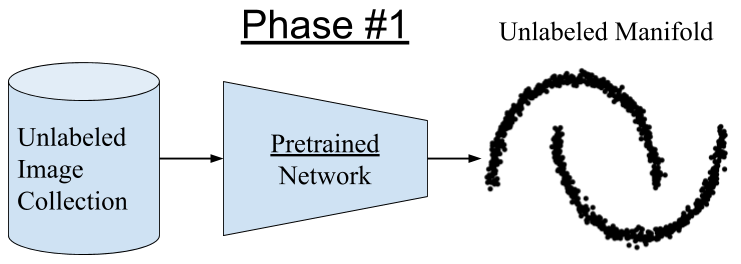Figure 1: A pretrained network embeds images into a manifold (feature space)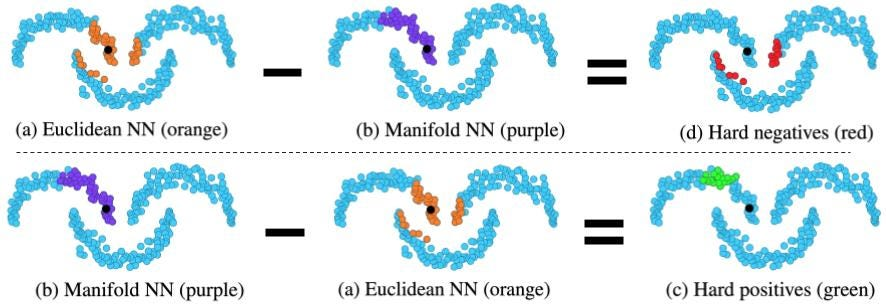Figure 2: Identify hard positive and negative images using the Euclidean and manifold nearest neighbors.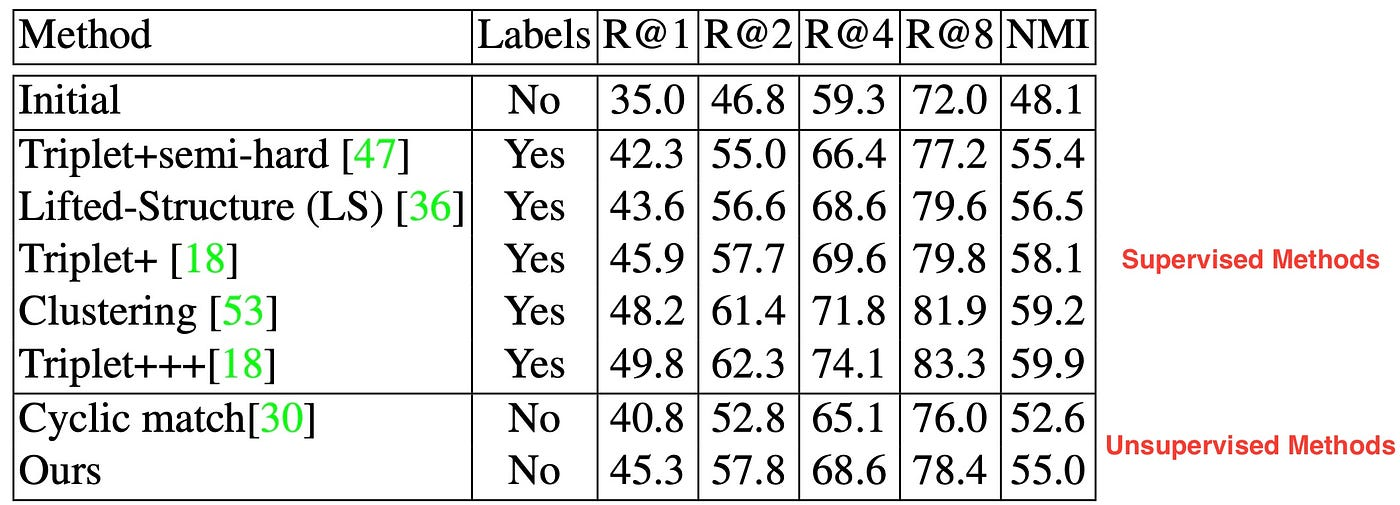Table 1: Recall@k and NMI on CUB-200–2011. All methods except for Ours and cyclic match  use ground-truth labels during training.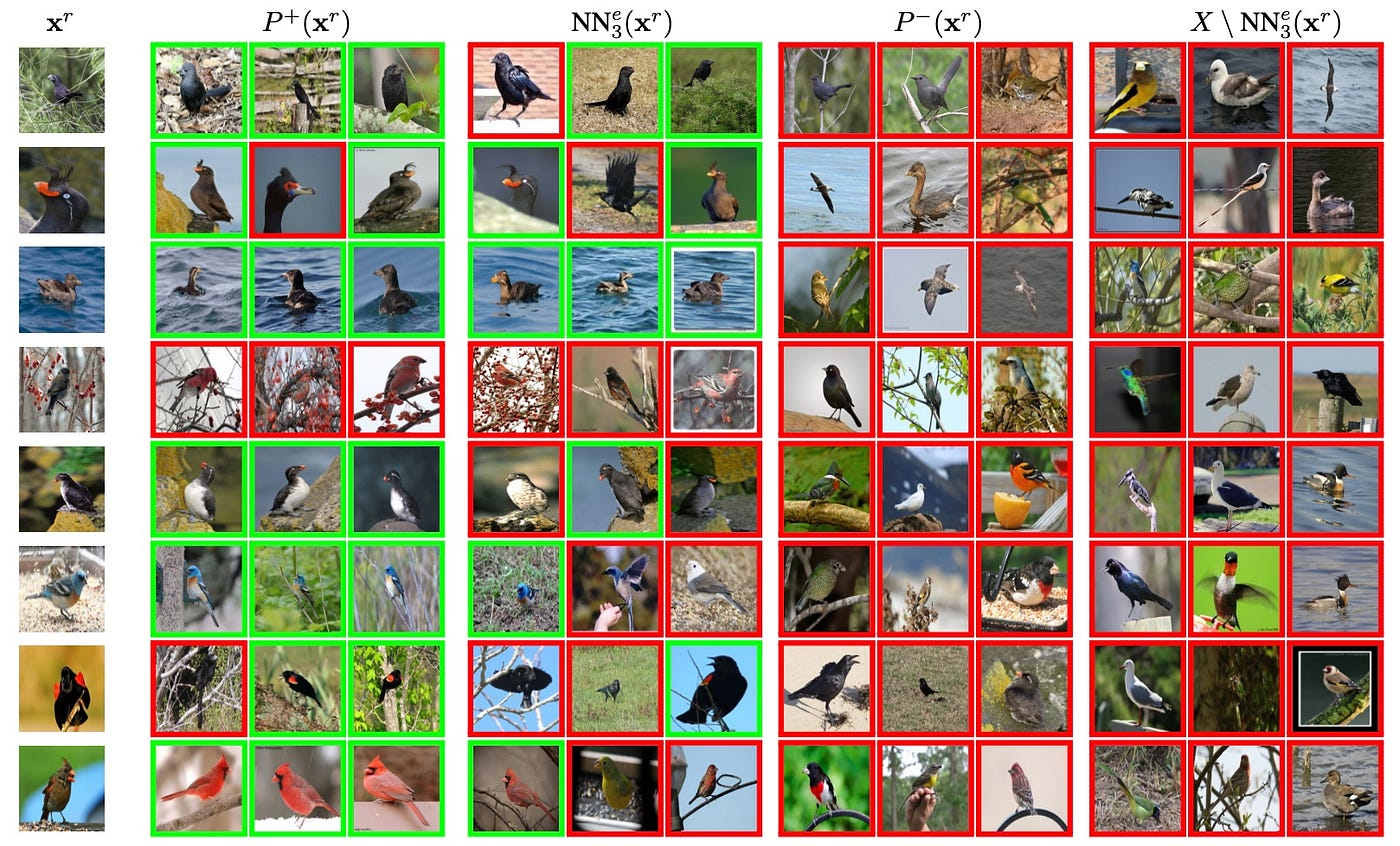Figure 3: Sample CUB-200-2011 anchor images (x^r), positive images from the proposed method (P^+(x^r)) and baseline (NN^e_3(x^r)), and negative images from the proposed method (P^−(x^r)) and baseline (X \ NN^e_3(x^r)). The baseline is Euclidean nearest neighbors and non-neighbors. Positive (negative) ground-truth framed in green (red). Labels are only used for qualitative evaluation and not during training. NN^e_3(x^r) indicates the three euclidean nearest neighbors to the anchor image x^r.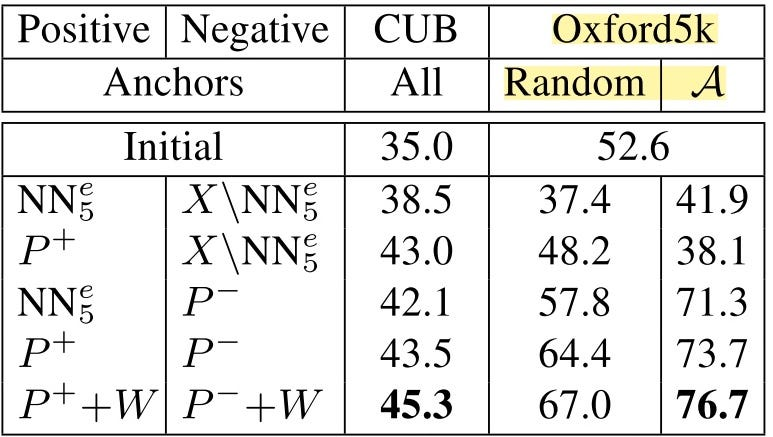Impact of choices of anchors and pools of positive and negative examples on Recall@1 on CUB-200–2011 and mAP on Oxford5k. Higher Recall/mAP is better. On CUB, all images are used as anchors, while on Oxford5K anchors are selected either at random or by the stationary probability distribution (A) approach. The positive and negative pools are formed by either the baseline with Euclidean nearest neighbors (NN^e) or the proposed hard-mining selection framework (P+ and P−).

--

--